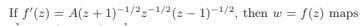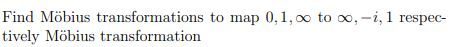Courses

Test

15 Questions MCQ Test Analytic Function | Test

Description
Attempt Test | 15 questions in 60 minutes | Mock test for Engineering preparation | Free important questions MCQ to study Analytic Function for Engineering Exam | Download free PDF with solutions
QUESTION: 1

Solution:
QUESTION: 2

Solution:
QUESTION: 3Solution:
QUESTION: 4

The linear fractional transformation T(z) = (z-i) / (z+1) maps the points

Solution:
QUESTION: 5

A linear fractional transformation is conformal at every point in its

Solution:
QUESTION: 6

The conformal mapping preserves both the magnitude and the ___ of an angle.

Solution:
QUESTION: 7Solution:
QUESTION: 8

If a complex function f is differentiable at point z, then f is

Solution:
QUESTION: 9

The function f(z) = z2 + z-1 is

Solution:
QUESTION: 10

The function f(z) = cos y - i sin y is

Solution:
QUESTION: 11

The function f(z) is analytic in a domain D and f(z) = c + i v(x,y), where c is a real constant. Then f is a _____ in D.

Solution:
QUESTION: 12

The function f(z) = (2-x)3 +i (y-1)3 is differentiable at z =

Solution:
QUESTION: 13

If the Cauchy-Riemann equations are true, then the derivative of f(z) is _____.

Solution:
QUESTION: 14

What is the value of the integer n if xn-yn is harmonic?

Solution:
QUESTION: 15

Find the harmonic conjugate of ex cos y + ey cos x + xy.

Solution:Use Code STAYHOME200 and get INR 200 additional OFF Use Coupon Code

Track your progress, build streaks, highlight & save important lessons and more!

Similar ContentRelated tests